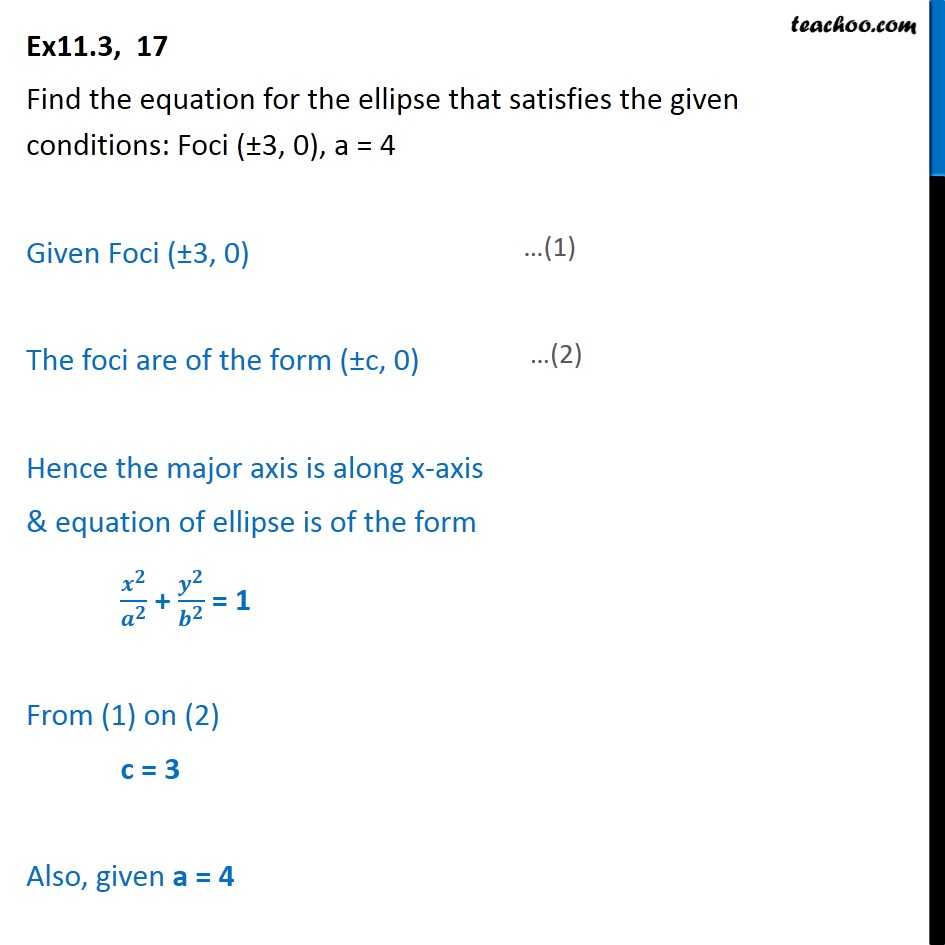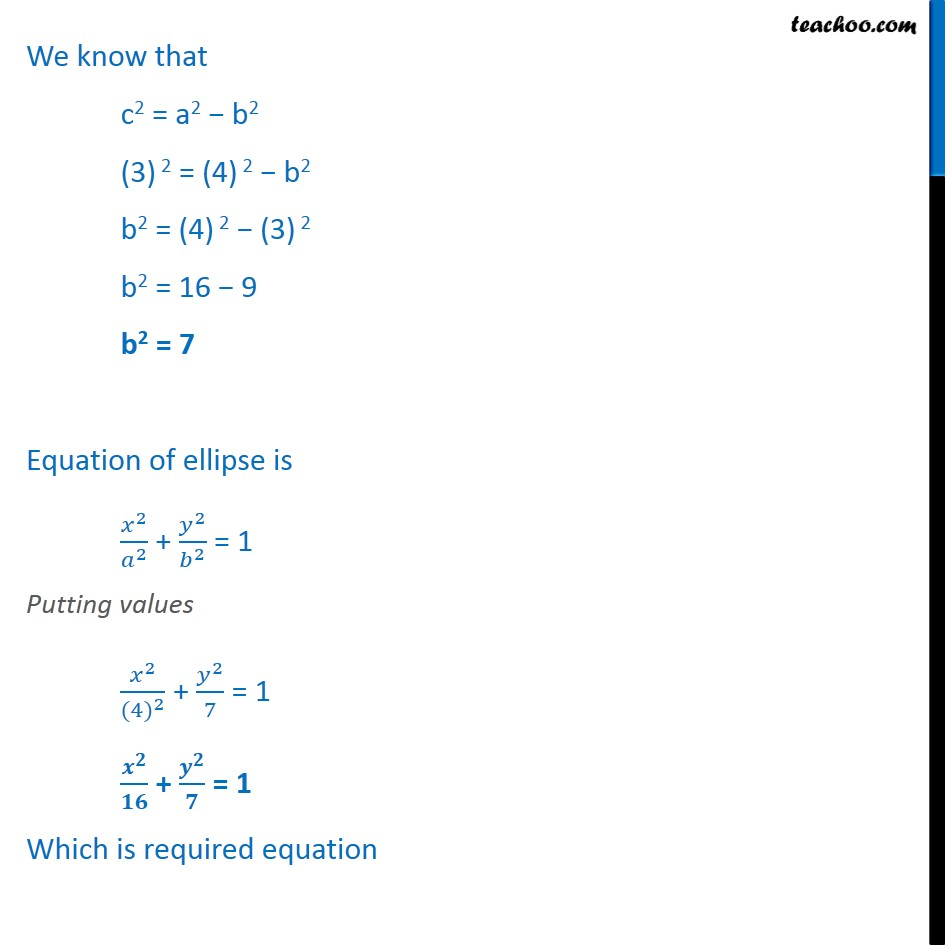Ex 10.3

Chapter 10 Class 11 Conic Sections
Serial order wiseLearn in your speed, with individual attention - Teachoo Maths 1-on-1 Class

### Transcript

Ex11.3, 17 Find the equation for the ellipse that satisfies the given conditions: Foci ( 3, 0), a = 4 Given Foci ( 3, 0) The foci are of the form ( c, 0) Hence the major axis is along x-axis & equation of ellipse is of the form + = 1 From (1) on (2) c = 3 Also, given a = 4 We know that c2 = a2 b2 (3) 2 = (4) 2 b2 b2 = (4) 2 (3) 2 b2 = 16 9 b2 = 7 Equation of ellipse is 2 2 + 2 2 = 1 Putting values 2 (4) 2 + 2 7 = 1 + = 1 Which is required equation